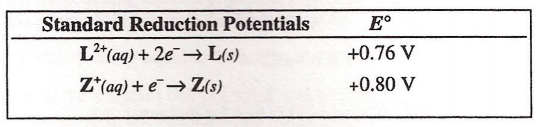Chemistry Practice Problems Redox Reaction Practice Problems Solution: Two metals are represented by the symbols L and Z....

🤓 Based on our data, we think this question is relevant for Professor Amunugama's class at EMU.

# Solution: Two metals are represented by the symbols L and Z. Which is the best oxidizing agent listed?(A) L(B) L2+(C) Z(D) Z+

###### Problem

Two metals are represented by the symbols L and Z. Which is the best oxidizing agent listed?

(A) L

(B) L2+

(C) Z

(D) Z+Redox Reaction

Redox Reaction

#### Q. Consider the following standard reduction potentials in acid solution:                                                             E°(V) Al3+ + 3e- →...

Solved • Sun Aug 28 2016 17:15:33 GMT-0400 (EDT)

Redox Reaction

#### Q. Given the overall redox reaction: Fe2+(aq) + Ce4+(aq) → Fe3+(aq) + Ce3+(aq)  Identify the two half-reactions. Oxidation half-reaction                 ...

Solved • Tue Aug 09 2016 10:12:13 GMT-0400 (EDT)

Redox Reaction

#### Q. Rank the following half reactions (1-5) in order of oxidizing ability, from strongest to weakest._______   K → K+ + e–                                ...

Solved • Tue May 17 2016 13:30:00 GMT-0400 (EDT)

Redox Reaction

#### Q. Which of these ions is the best reducing agent?  Standard Reduction Potentials                   E°      Fe 3+ (aq) + e– → Fe 2+ (aq)               ...

Solved • Tue Apr 26 2016 15:35:27 GMT-0400 (EDT)